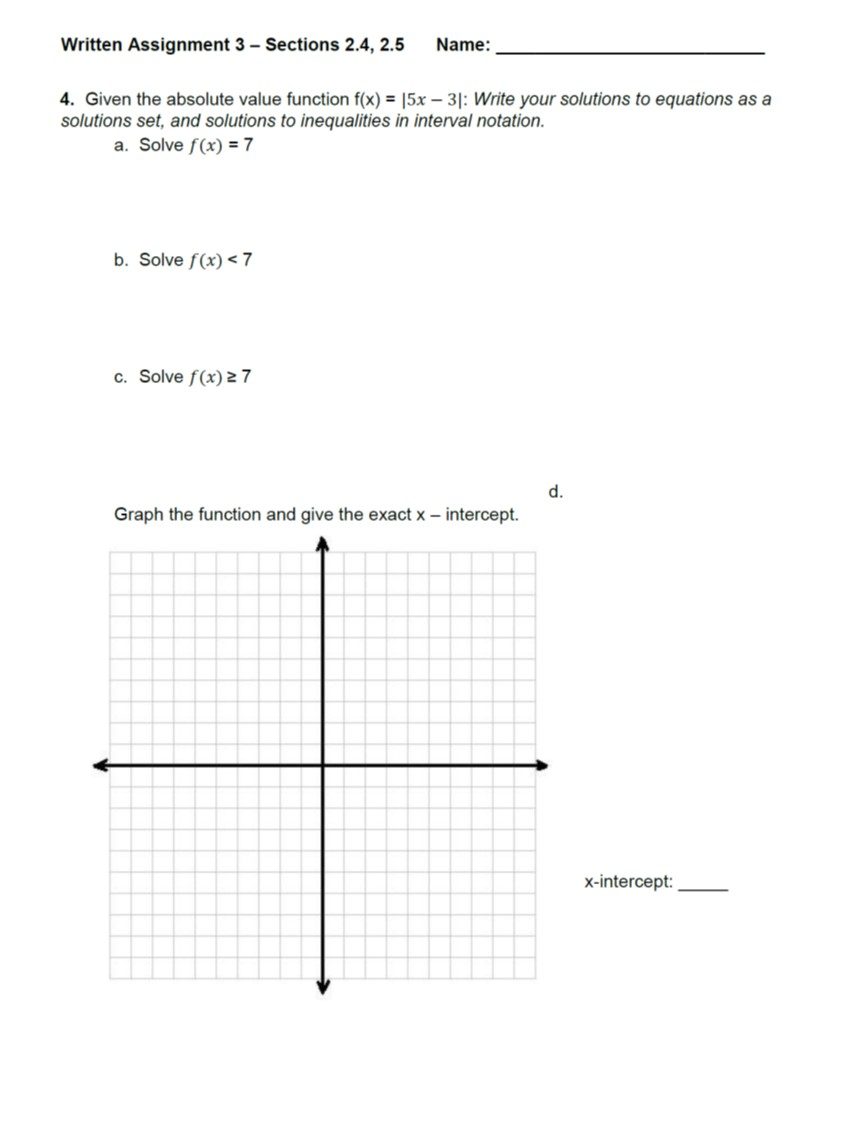Written Assignment 3 Sections 2.4, 2.5 Name:4. Given the absolute value function f(x) = 15x-31: Write your solutions to equations as asolutions set, and solutions to inequalities in interval notation.a. Solve f(x)=7b. Solve f(x) < 7c. Solve f(x) 27d.Graph the function and give the exact x - intercept.x-intercept:

Question

Dears,
Thankshelp_outlineImage TranscriptioncloseWritten Assignment 3 Sections 2.4, 2.5 Name: 4. Given the absolute value function f(x) = 15x-31: Write your solutions to equations as a solutions set, and solutions to inequalities in interval notation. a. Solve f(x)=7 b. Solve f(x) < 7 c. Solve f(x) 27 d. Graph the function and give the exact x - intercept. x-intercept: fullscreen
Step 1

To solve the given equations and inequalities involving the absolute value function graphically

Step 2

The   function f(x)=|5x-3| is defined by the conditions shown.

Step 3

So, the graph consists of parts of two straight lines: y=5x-3 for x greater than or equal to 0.6, y=-(5x-3) if x is less than 0.6. Note th...

Want to see the full answer?

See Solution

Want to see this answer and more?

Our solutions are written by experts, many with advanced degrees, and available 24/7

See Solution
Tagged in

Algebra